### Rui Benfeitas, Scilifelab, NBIS National Bioinformatics Infrastructure Sweden¶

rui.benfeitas@scilifelab.se

#### Abstract¶

In this notebook we will examine several properties in preparing our data for integration. However, it should be noted that a correct preparation of your dataset will depend on your data's technology among other factors.
We start from an overview of our dataset, followed by removing redundant or uninformative features. We then tackle different methods of data imputation, outlier detection, and a quick data profiling of feature behavior and quality. Finaly, we look into data transformation, including rescaling, normalization and batch correction.

This notebook should be seen as a reminder to several factors that we need to consider before downstream analyses, and there are any alternative approaches to tackle a specific problem such as missingness or outlier detection. As such, this guide should not be seen as an exhaustive benchmarking notebook, nor should it be taken as replacement for dedicated QC and pre-processing methods or pipelines. For more information, refer to NBIS workshops or Scilifelab courses.

# Preamble¶

Before starting, it is crucial that we have as much information about our dataset as possible, and that we have a good idea of the analyses that we will perform afterwards. Some of the questions to bear in mind before starting:

• What is your dataset size?
Different data preparation approaches and downstream analyses require prior knowledge of the number of samples and their distribution given your experimental design. For instance, should there be batch effects perhaps it is a good idea to stratify your samples if the number of samples per strata allows for it. If your dataset size is big perhaps you can consider deep learning techniques in your analyses. However, we should always be careful with the curse of dimensionality.
• What sample metadata is available? (e.g. clinical variables, sample run order, plate identifier, sequencing depth).
By using all the relevant metadata available, you will be able to understand how best to prepare your dataset and check for deviations from the sample population. You will be able to check for outliers with respect to the sample groups, or check if there are any confounding factors that have biased your data. If your dataset is strongly confounded or affected by outliers, any downstream analysis will reflect these technical differences rather than biological variability. If you are aware of confounding factors, you may control for them in your modeling design, stratify your samples, or rescale your data to account for them.
• What methods will you use for downstream analysis?
The choice of downstream analysis will depend on your data, but will also imply that your data needs to be prepared in the correct format. Does your data need to be scaled? Do you need absolute quantifications or do relative quantifications suffice?
• Does it make sense to rescale features/samples?
If you are considering whether or not to rescale your data, you should think about your data types and experiment design. Perhaps you want to rescale your data at the sample level in order to remove batch effects, at the risk of removing biologically-important sources of variability. Or perhaps your dataset contains data from different biological sources (e.g. metabolomic and transcriptomic data), so you should question whether it makes sense to compute the metrics required for sample-wide normalization. Or perhaps you want to assess whether these different data sources may have a similar contribution despite their widely different sample scales.

Being able to answer these questions before starting to clean and prepare your data enables better informed decisions throughout. We will examine some of these questions throughout this notebook.

Currently, the topics below are discussed in this notebook. Note however that while this represents a general workflow, it is often necessary to step back and re-examine different parameters of the data as we analyse it.

In :
from graphviz import Digraph

g = Digraph('G')
g.attr(rankdir='same', compound='true', fontstyle='Verdana',ranksep = '1', labelloc="t",

with g.subgraph(name='cluster0') as c:
c.attr(label='Data cleaning', fontsize='12', labeljust='left', href='#data-cleaning', fontcolor='blue')
c.node_attr.update(style='filled', color='white')
c.node('uninformative data', fontcolor='gray', fontsize='10')
c.node('redundant data', fontcolor='gray', fontsize='10')

with g.subgraph(name='cluster1') as c:
c.attr(label='Imputation', fontsize='12', labeljust='left', href='#handling-missing-values', fontcolor='blue')
c.node_attr.update(style='filled', color='white')
c.node('mean / median', fontcolor='gray', fontsize='10')
c.node('minimum / 0', fontcolor='gray', fontsize='10')
c.node('KNN', fontcolor='gray', fontsize='10')
c.node('Bayesian Ridge', fontcolor='gray', fontsize='10')
c.node('Decision Tree', fontcolor='gray', fontsize='10')

with g.subgraph(name='cluster2') as c:
c.attr(label='Anomaly detection', fontsize='12', labeljust='left', href='#anomaly-detection', fontcolor='blue')
c.node_attr.update(style='filled', color='white')
c.node('Data ranges', fontcolor='gray', fontsize='10')
c.node('HDBSCAN', fontcolor='gray', fontsize='10')
c.node('LOF', fontcolor='gray', fontsize='10')
c.node('Isolation Forest', fontcolor='gray', fontsize='10')
c.node('One-class SVM', fontcolor='gray', fontsize='10')

with g.subgraph(name='cluster3') as c:
c.attr(label='Data Profiling', fontsize='12', labeljust='left', href='#data-profiling', fontcolor='blue')
c.node_attr.update(style='filled', color='white')
c.node('DABL', fontcolor='gray', fontsize='10')
c.node('Pandas Profiling', fontcolor='gray', fontsize='10')
c.node('Dimensionality Reduction', fontcolor='gray', fontsize='10')

with g.subgraph(name='cluster4') as c:
c.attr(label='Data Transformation', fontsize='12', labeljust='left', href='#data-transformation', fontcolor='blue')
c.node_attr.update(style='filled', color='white')
c.node('Scaling', fontcolor='gray', fontsize='10')
c.node('Standardization', fontcolor='gray', fontsize='10')
c.node('Quantile normalization', fontcolor='gray', fontsize='10')
c.node('Normalization', fontcolor='gray', fontsize='10')
c.node('ComBat', fontcolor='gray', fontsize='10')

g.edge('Raw data', 'Data overview', fontsize='12')
g.edge('Data overview', 'uninformative data', lhead='cluster0', fontsize='12')
g.edge('Pandas Profiling', 'Quantile normalization', ltail='cluster3', lhead='cluster4')
g.edge('Quantile normalization', 'Integration', ltail='cluster4')

g.node('Raw data', shape='box', fontsize='12')
g.node('Data overview', shape='box', fontsize='12', href='#dataset-overview', fontcolor='blue')
g.node('Integration', shape='box', fontsize='12')

g

Out:

# dataset overview¶

In :
############
# Preamble #
############

### General packages
import os, matplotlib, sklearn, re, warnings
import pandas as pd
import seaborn as sb
import numpy as np
import scipy as sp
import matplotlib.pyplot as plt
import statsmodels
from statsmodels.stats import multitest

### Dim reduction
from pca import pca
import umap
import umap.plot

### Data imputation
from sklearn.experimental import enable_iterative_imputer
from sklearn.pipeline import make_pipeline
from sklearn.impute import SimpleImputer, KNNImputer, IterativeImputer
from sklearn.tree import DecisionTreeRegressor
from sklearn.linear_model import BayesianRidge
from sklearn.neighbors import KNeighborsRegressor
from sklearn.model_selection import cross_val_score, RepeatedStratifiedKFold, RepeatedKFold

### Integrity checks
# Outlier detection
import hdbscan
from sklearn.ensemble import IsolationForest
from sklearn.svm import OneClassSVM

# Data profiling
import pandas_profiling
import dabl

### batch effect removal
from combat.pycombat import pycombat

### Other settings
warnings.simplefilter("ignore", category=sklearn.exceptions.ConvergenceWarning)
np.random.seed(0)

%matplotlib inline


This dataset comprises 57 samples from 3 groups of patients (healthy, disease group1, and disease group2). For each sample, we have the patient gender and the categorical severity from low (1) to high (4). We have gene expression data for about 7k genes.

In :
## Data importing

In :
## 7029 genes, 59 columns including 2 for id mapping
gex.shape

Out:
(7029, 59)
In :
gex.head()

Out:
ensembl gene_description sample_1 sample_10 sample_11 sample_12 sample_13 sample_14 sample_15 sample_16 ... sample_54 sample_55 sample_56 sample_57 sample_58 sample_59 sample_6 sample_7 sample_8 sample_9
gene
OR1S1 ENSG00000280204 Olfactory receptor family 1 subfamily S member... 0.0 0.0 0.000 0.0 0.0 0.0 0.0 0.0 ... 0.0 0.0 0.0 0.0 0.0 0.0 0.000 0.0 NaN 0.0
MBD3L4 ENSG00000205718 Methyl-CpG binding domain protein 3 like 4 0.0 NaN 0.000 0.0 0.0 0.0 0.0 0.0 ... 0.0 0.0 0.0 0.0 0.0 0.0 0.000 NaN NaN 0.0
DEFB115 ENSG00000215547 Defensin beta 115 0.0 0.0 0.000 0.0 0.0 NaN NaN 0.0 ... 0.0 0.0 0.0 0.0 0.0 0.0 0.032 0.0 0.0 0.0
OR2B3 ENSG00000204703 Olfactory receptor family 2 subfamily B member 3 0.0 0.0 0.001 0.0 0.0 0.0 0.0 0.0 ... 0.0 0.0 0.0 0.0 NaN NaN 0.000 0.0 0.0 0.0
IFNA13 ENSG00000233816 Interferon alpha 13 0.0 0.0 0.000 0.0 0.0 NaN 0.0 NaN ... 0.0 0.0 0.0 0.0 0.0 0.0 0.000 NaN NaN 0.0

5 rows × 59 columns

In :
metadata.head()

Out:
group gender severity
samples
sample_1 group1 Male 2.0
sample_2 group1 Male 3.0
sample_3 group1 Female 4.0
sample_4 group1 Female 2.0
sample_5 group1 Male 1.0

Number of samples per group.

In :
metadata.groupby('group')['gender'].agg('count')

Out:
group
control     7
group1     35
group2     15
Name: gender, dtype: int64

Number of samples per disease severity (1 - least severe, 4 - most severe).

In :
metadata.fillna('no_disease').groupby('severity')['gender'].agg('count')

Out:
severity
1.0            1
2.0           12
3.0           20
4.0           17
no_disease     7
Name: gender, dtype: int64

We will further add the group information to the columns names (groups: g1, g2 and ct) dataset names to make it easier later, and use a dataframe only with gene expression values.

In :
metadata.loc[:,'group_sample']=metadata.index+'.'+metadata.group.str.replace('(roup)','', regex=True).str.replace('control','ct')

gex_values=gex.loc[:, ~gex.columns.isin(['ensembl','gene_description'])] #only values

Out:
sample_1.g1 sample_10.g1 sample_11.g1 sample_12.g1 sample_13.g1 sample_14.g1 sample_15.g1 sample_16.g1 sample_17.g1 sample_18.g1 ... sample_54.ct sample_55.ct sample_56.ct sample_57.ct sample_58.ct sample_59.ct sample_6.g1 sample_7.g1 sample_8.g1 sample_9.g1
gene
OR1S1 0.0 0.0 0.000 0.0 0.0 0.0 0.0 0.0 0.0 0.0 ... 0.0 0.0 0.0 0.0 0.0 0.0 0.000 0.0 NaN 0.0
MBD3L4 0.0 NaN 0.000 0.0 0.0 0.0 0.0 0.0 0.0 0.0 ... 0.0 0.0 0.0 0.0 0.0 0.0 0.000 NaN NaN 0.0
DEFB115 0.0 0.0 0.000 0.0 0.0 NaN NaN 0.0 0.0 0.0 ... 0.0 0.0 0.0 0.0 0.0 0.0 0.032 0.0 0.0 0.0
OR2B3 0.0 0.0 0.001 0.0 0.0 0.0 0.0 0.0 0.0 0.0 ... 0.0 0.0 0.0 0.0 NaN NaN 0.000 0.0 0.0 0.0
IFNA13 0.0 0.0 0.000 0.0 0.0 NaN 0.0 NaN 0.0 0.0 ... 0.0 0.0 0.0 0.0 0.0 0.0 0.000 NaN NaN 0.0

5 rows × 57 columns

# data cleaning¶

## repeated or uninformative data¶

Before starting cleaning your dataset, one of the things to check is whether there are any duplicated features or samples.
For instance, if this was a proteomic dataset, how would you deal with two isoforms of the same protein? And how would you do it if you had a dataset where multiple features (e.g. probes) are associated with the same gene? You could consider

1. Dropping repeated features (thus risking losing potentially important information);
2. Summarizing all related features by computing means or medians (which could dim potentially important biological variation for different features); or
3. Modeling all sepparately (thus keeping all identified variation, but risking having your downstream analyses biased by features that display higher number of duplications).

Note, however, that attention needs to be taken in the case of crossover studies. Choosing the best solution will depend on your dataset and objective.

In :
if(gex.index.duplicated().any()):
print('Duplicated genes found.')
else:
print('No duplicates found.')

No duplicates found.

In :
if(gex.columns.duplicated().any()):
print('Duplicated columns found.')
else:
print('No duplicates found.')

No duplicates found.


On the other hand, you may have uninformative features in your dataset. For instance, we may have invariant features (those whose variance $\sigma^2$ is null), or those that are null throughout. The following plots represent the population density distribution of feature variance (left) and row sums (right).

In :
fig,ax =plt.subplots(ncols=2, figsize=(16,5))
ax=ax.flatten()
sb.kdeplot( gex_values.var(1, skipna=True), ax=ax)
ax.set_title('feature variability')
ax.set_xlabel('variance')
sb.kdeplot( gex_values.sum(1, skipna=True), ax=ax, label='this')
ax.set_title('null features')
ax.set_xlabel('row sum')
plt.show()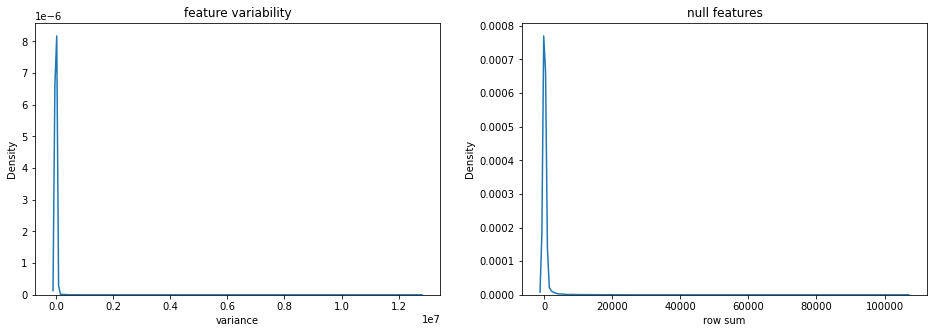These uninformative features will add nothing to any sample vs sample or feature vs feature comparisons that we may be interested. We will remove them from our dataset as they contribute with no information to our downstream analyses.

In :
## flagging uninformative features for removal
uninformative_features=gex_values.index.values[(gex_values.sum(1, skipna=True) == 0) | (gex_values.var(1, skipna=True) == 0)]


Bear in mind that we may still have other kinds of uninformative features, such as those that are basically adding noise and do not really help in specific tasks such as sample clustering for instance. You may be interested in looking at this feature selection tutorial, or the respective lesson content on Github.

## handling missing values¶

Missing values may be problematic if very prevalent in our dataset. It is also important to understand that missingness may have a biological rather than technical meaning: is a feature absent in a sample because of instrument detection limits, or because it is not present in a sample? Assuming the former, we have two ways of dealing with missing data:

• Dropping - never desirable to drop precious (and expensive) data, but sometimes unavoidable;
• Imputing - missing value imputation can be done in different ways, and should be done carefully or it may introduce spurious bias in the data.

### Dropping¶

If our data has many missing values, one should question the validity of any hypotheses generated with its samples - we do not want to predict biomarkers or sample strata based on missing/imputed data. For this reason, we examine both features and samples for missing data. However, note that by removing features you are reducing the degrees of freedom of your analysis and potentially losing biologically important information.

We start by examining at the sample level. We see that samples have about ~20% missing values, except for three samples which have a very high (>60%) number of missing values. We will drop these from further analysis.

In :
### NA ratios per sample
NAs_sample_ratios=gex_values.isna().sum()/gex_values.index.shape

fig,ax=plt.subplots(figsize=(16,3))
sb.barplot(
x=np.arange(0,gex_values.shape),
y=NAs_sample_ratios.values,
ax=ax
)
plt.xlabel('sample');
plt.ylabel('ratio of NAs');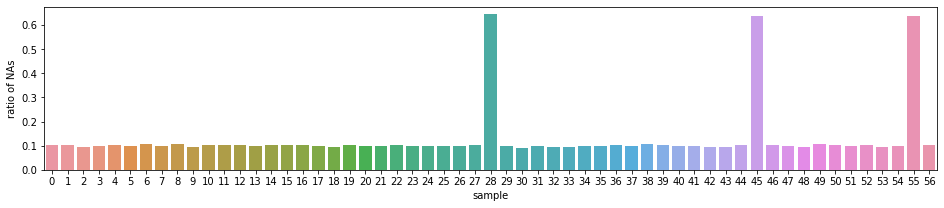Note that because of our sample numbers per group above, (control = 7, group1 = 35, group2 = 15), we should question at this point whether the number of NAs is imbalanced and one group shows more than the others. However, we see that the mean and distributions of NAs between samples is fairly similar between groups. The "outliers" shown in the boxplots are those 3 samples showing very high NA abundance above, all of which belong to group1.

In :
### NA by sample groups

### Mean per group
# NAs_sample_groups.groupby('group')['NA_ratio'].agg('mean')

fig,ax=plt.subplots(ncols=2, figsize=(12,5));
ax=ax.flatten();
## Full plot
g=sb.boxplot(
data=NAs_sample_groups,
x='group',
y='NA_ratio', hue='group',
ax=ax
);
g.legend_.remove()
ax.title.set_text('Full')

## No outliers
sb.boxplot(
data=NAs_sample_groups,
x='group', y='NA_ratio', hue='group',
showfliers=False,
ax=ax
)
ax.title.set_text('Without outliers')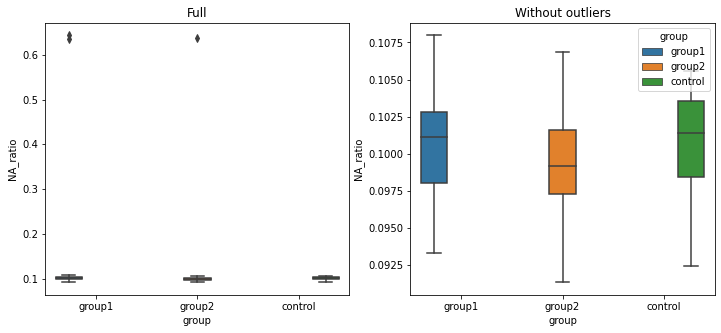We also examine missingness at the gene level. We see that most genes have very low number of NAs (1st quartile 11%, 3rd quartile 16%, mean 13%). But for some genes, they display a very high number of missing values (max 30%).

In :
# NAs per gene
NAs_gene_ratios=(gex_values.isna().sum(1)/gex_values.columns.shape)

##### Description of missignness at gene level:
#NAs_gene_ratios.describe()

## Because we have >7000 genes, we plot their distributions as histogram and kernel density plots.
sb.displot(NAs_gene_ratios)
plt.xlabel('ratio of NAs');
plt.ylabel('kernel histogram');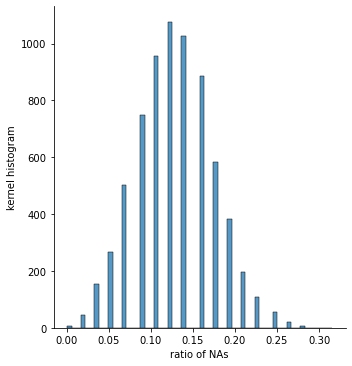We will remove the 3 problematic samples above, and genes displaying >15% missing values (i.e. NAs in more than 8.5 samples).

In :
## problematic samples show NA> 60%
problematic_samples=NAs_sample_groups.index[NAs_sample_groups.NA_ratio>0.6]

## problematic genes
problematic_genes=NAs_gene_ratios.index[NAs_gene_ratios>0.15]

## Filtering
# recall that we selected uninformative features above based on variance and row sums
gex_filtered=gex_values.copy().loc[
(~gex_values.index.isin(np.append(problematic_genes, uninformative_features))),]
gex_filtered=gex_filtered.loc[:,  ~gex_values.columns.isin(problematic_samples)]

## 4767 genes, 54 samples
gex_filtered.shape

Out:
(4767, 54)

### Imputation¶

We can employ different strategies for dealing with the missing values. It may be useful to flag missing values, as this will permit identifying and saving which features/samples have missing values to examine the influence of missingness and imputation. There are different types of imputation:

Single value imputation - Imputation by one plausible value. Examples:

• Mean / Median / Minimum / 0 - filling data based on a constant.
• KNN - filling data based on most similar neighbours

Multiple imputation - The distributions of the observed data are used to estimate multiple values that reflect the uncertainty around the true value, and pooled to predict the imputed value.

• Bayesian Ridge regression - regularized linear regression imputation
• Decision tree regression - non-linear regression imputation

Note that the choice of imputation method may have dramatic effects on our data behavior, and could bias any analyses done with the data. For instance, how would we expect samples to cluster if they have a high number of 0-imputed features compared to samples with very few or no 0-imputed features?

Scikitlearn and autoimpute present many imputation strategies. Here, we'll explore some of the imputation strategies through scikitlearn.

#### Mean / Median / Minimum / 0¶

We could impute based on row (gene) mean, median, minimum or 0. Note that considering these imply different assumptions about the cause of missingness: by considering mean or median, we are assuming that value missingness is random; by considering minimum, minimum$*$0.95 or 0, we are assuming that quantifications are missing due to instrument sensitivity for instance. Choosing one or the other will thus depend on the technology used and on what we know about missigness in our data (further discussed at the end of this section).

Note that using mean, median, minimum or 0 does not account for correlations between features, and may severely bias the data.

We start by doing 0-imputation, or based on row-wise mean, median or minimum.

In :
# impute values as 0
imputed_GEX_0=gex_filtered.copy().fillna(0)

# impute values based on row means
imputed_GEX_rowMEAN=pd.DataFrame(
SimpleImputer(strategy='mean').fit_transform(gex_filtered.T).T,
index=gex_filtered.index, columns=gex_filtered.columns)

# row median
imputed_GEX_rowMEDIAN=gex_filtered.copy().T.fillna(gex_filtered.median(1)).T

#row min
imputed_GEX_rowMIN=gex_filtered.copy().T.fillna(gex_filtered.min(1)).T


Note however that different groups of samples are present, so perhaps a more accurate way to account for between-group variability is to impute based on group-specific metrics. We do so below with mean, median, minimum.

In :
def impute_by_group(impute_strategy):
"""
Imputes our dataset based on group-wise information.

impute_strategy      string. options: 'min','min*0.95', or any argument to strategy in SimpleImputer.

Returns:
imputed dataframe
"""
group1=gex_filtered.copy().loc[:, gex_filtered.columns.str.contains('\.g1$')] #group1 group2=gex_filtered.copy().loc[:, gex_filtered.columns.str.contains('\.g2$')] #group2
groupCT=gex_filtered.copy().loc[:, gex_filtered.columns.str.contains('\.ct$')] #controls # SimpleImputer does not include "min" as argument, so we compute it here ourselves if(impute_strategy in ['min', 'min*0.95']): imputed_g1=group1.T.fillna(value=group1.min(1, skipna=True)).T imputed_g2=group2.T.fillna(value=group2.min(1, skipna=True)).T imputed_ct=groupCT.T.fillna(value=groupCT.min(1, skipna=True)).T # mean and median are valid arguments to SimpleImputer(strategy=...) elif(impute_strategy in ['mean', 'median']): #values imputed from samples in group1 imputed_g1=pd.DataFrame( SimpleImputer(strategy=impute_strategy).fit_transform(group1.T).T, index=group1.index, columns=group1.columns) #values imputed from samples in group2 imputed_g2=pd.DataFrame( SimpleImputer(strategy=impute_strategy).fit_transform(group2.T).T, index=group2.index, columns=group2.columns) #values imputed from samples in control imputed_ct=pd.DataFrame( SimpleImputer(strategy=impute_strategy).fit_transform(groupCT.T).T, index=groupCT.index, columns=groupCT.columns) else: raise ValueError('Incorrect argument for imput_strategy. Options: min, min*0.95, mean, or median.') output=imputed_g1.join(imputed_g2).join(imputed_ct) #joining imputed datasets return(output) ## Imputation by group mean in each group imputed_GEX_groupMEAN=impute_by_group('mean') ## Imputation by group median in each group imputed_GEX_groupMEDIAN=impute_by_group('median') ## Imputation by minimum in group imputed_GEX_groupMIN=impute_by_group('min') ## Imputation by min*0.95 imputed_GEX_groupMIN95=impute_by_group('min*0.95')  #### KNN imputation¶ We may also fill data based on the K-nearest neighbour (KNN) similarity. In this approach, the mean among the k nearest neighbors is used after training a KNN model. Note that the key hyperparameter here is the number of neighbours to consider. An often used good choice for$ k = \sqrt(\text{sample number}) = \sqrt(57) \approx 8$. We will perform a grid search on odd numbers for$1 \leq k \leq 20$. We start by encoding class labels as numbers, and identify the optimum k by considering that all neighbors within a boundary have the same weight (weights = uniform), and use Euclidean distance as the metric to quantify distance between neighbouring points (metric = euclidean). We use sample groups (g1, g2, ct) as class labels since KNN is a supervised technique, and use 5-fold crossvalidation. We use accuracy as score for training and testing data. We should bear in mind that data standardization may be required (as we further discuss in another section below), but we skip it for now for simplicity sake. In : # Encode class labels (groups) into integers to use for the estimators below class_labels=sklearn.preprocessing.LabelEncoder().fit_transform(gex_filtered.columns.str.replace('.+\.','') ) # Standardized gene expression std_gex=pd.DataFrame(sklearn.preprocessing.StandardScaler().fit_transform(gex_filtered.T), index=gex_filtered.columns, columns=gex_filtered.index).T ### We imput NA with random values in the same range of the min np.random.seed(0) random_df=np.random.uniform( low=std_gex.min(), high=std_gex.max(), size=std_gex.shape) # impute the values where NAs are found randomly_imputed=std_gex.copy() randomly_imputed[randomly_imputed.isna()]=random_df # standardizing mean-imputed data std_gex_groupmean=pd.DataFrame( sklearn.preprocessing.StandardScaler().fit_transform(imputed_GEX_groupMEAN.T), index=imputed_GEX_groupMEAN.columns, columns=imputed_GEX_groupMEAN.index) # gridsearch to find optimum k based on randomly imputed data knn=sklearn.neighbors.KNeighborsClassifier(metric='euclidean') grid_pars={ 'n_neighbors': range(1, 21), ### uncomment the following lines to tune the weights and metric hyperparameters ### remember to remove these arguments from the function knn above # 'weights': ['uniform','distance'], # 'metric':['euclidean','manhattan'] } #5-fold CV, preserving label distribution/fold, repeated 20 times skfold=RepeatedStratifiedKFold(n_splits=5, n_repeats=20) knn_gridcv=sklearn.model_selection.GridSearchCV( knn, grid_pars, cv=skfold, scoring={'Accuracy': sklearn.metrics.make_scorer(sklearn.metrics.accuracy_score)}, refit='Accuracy', return_train_score=True ) knn_results_random=knn_gridcv.fit( randomly_imputed.T.values, class_labels) print(f''' Random-imputed data - optimum k: {knn_results_random.best_params_['n_neighbors']} \tAccuracy (train): {np.round(knn_results_random.cv_results_['mean_train_Accuracy'][knn_results_random.best_index_],2)} \tAccuracy (test): {np.round(knn_results_random.cv_results_['mean_test_Accuracy'][knn_results_random.best_index_],2)} ''')  /Users/rui.benfeitas/opt/miniconda3/envs/env_data_preprocess/lib/python3.7/site-packages/ipykernel_launcher.py:2: FutureWarning: The default value of regex will change from True to False in a future version.   Random-imputed data - optimum k: 4 Accuracy (train): 0.83 Accuracy (test): 0.76  We next plot the accuracy of the training and testing sets for a KNN imputation whose optimum k = 4 was determined after random imputation. The following plot marks in the test set accuracy at k = 2, as well as$ k = \sqrt(\text{sample number}) = \sqrt(57) \approx 8$. In : ######################################## # Best results of KNN run knn_random_df_output=pd.DataFrame(knn_results_random.cv_results_); best_index=knn_results_random.best_params_['n_neighbors'] best_score=knn_results_random.best_score_ ######################################## # Imputation by KNN for later use imputed_GEX_KNN=pd.DataFrame( KNNImputer(n_neighbors=best_index).fit_transform(std_gex.T), index=std_gex.columns, columns=std_gex.index).T # Imputation by KNN with k = 8 imputed_GEX_KNN8=pd.DataFrame( KNNImputer(n_neighbors=8).fit_transform(std_gex.T), index=std_gex.columns, columns=std_gex.index).T ######################################## # Plotting fig,ax=plt.subplots(figsize=(10,6)) # model score sb.lineplot( data=knn_random_df_output, x='param_n_neighbors', y='mean_train_Accuracy', color='black', label='random imputation (train)') sb.lineplot( data=knn_random_df_output, x='param_n_neighbors', y='mean_test_Accuracy', color='black', label='random imputation (test)'); ax.fill_between(range(1,21), knn_random_df_output['mean_test_Accuracy'] - knn_random_df_output['std_test_Accuracy'], knn_random_df_output['mean_test_Accuracy'] + knn_random_df_output['std_test_Accuracy'], alpha=0.1, color='black') # best score ax.annotate("%0.3f" % best_score, (best_index+0.3, best_score + 0.005), color='black', size='12') ax.plot([best_index]*2,[0,best_score],color='black', marker='x', markeredgewidth=5, ms=12) # k = 8 ax.plot(*2,[0,knn_results_random.cv_results_['mean_test_Accuracy']],color='gray', marker='x', markeredgewidth=5, ms=12) #k = 8, random imputed ax.annotate("%0.3f" % knn_results_random.cv_results_['mean_test_Accuracy'], (8, knn_results_random.cv_results_['mean_test_Accuracy']-0.035), color='gray', size='12') ax.lines.set_linestyle("--") ax.lines.set_linestyle("--") ax.legend().get_lines().set_linestyle("--") ax.set(ylabel='Mean accuracy', ylim=(0.5,1.01)); ax.set_xticks(np.arange(1,20,2)); plt.title('Mean crossvalidation accuracy');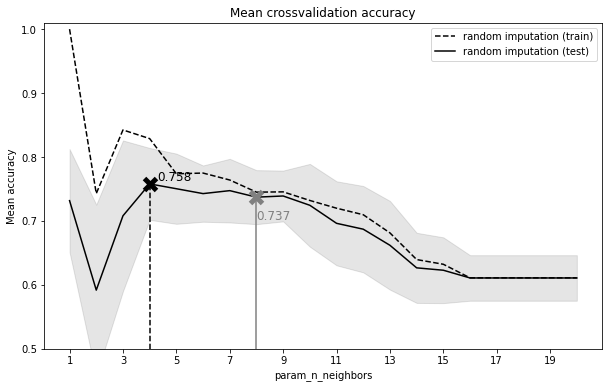Based on the accuracy of the test data we find an optimum$ k = 4$(black cross above), though a$ k = 8$achieves a similar accuracy (gray cross above). Our selection of best k is done based on the test data and not on the training data, though the latter has substantially higher accuracy. Note that we get a perfect training accuracy for k = 1 but poor testing accuracy on training data (why is this so?). We also found that k = 1 has a similarly high acuracy in the testing set. However, we should be careful in using very low k as these can usually can be noisy and lead to more "complex" models in terms of the decision boundaries. In turn, larger k will usually present smoother decision boundaries, implying lower variance but higher bias. #### Imputation with Decision Trees and on Bayesian Ridge Regression¶ For multivariate imputation we could apply a similar approach, where a model is built for each feature with missing values, modeled after the n-closest (or all) known features. The regressed model is then used to predict the missing values. This is done iteratively for each feature, and repeated up to a maximum number of iterations. Decision Trees and Bayesian Ridge Regression are multivariate imputation strategies. Decision trees are used for a non-linear imputation after regressing features on complete data. In Bayesian ridge regression, features are imputed based on the distributions of the n most similar features, taking advantage of the$L_2$regularization to control for overfitting assuming normally distributed model parameters as priors, and using those to estimate distributions of possible parameter values for the model (as opposed to single values computed through frequentist linear regression). For each round of imputation of a feature, a stopping criterion is defined as $$\frac{abs( max( X_t - X_{t-1}))}{abs(max(X_{\text{known_vals}}))} < tol$$ where$X_t$is the value of the feature at iteration$t$, and$tol$is a predefined value (default tol = 0.001). This is done for each feature in an iterative fashion, repeated for max_iter imputation rounds until the stopping criterion is reached, and the results of the final imputation round are returned. For both approaches, we specify that minimum and maximum imputed values must be within the value range of the standardized dataset. We increase the maximum number of iterations and the 10 closest features (rather than the entire dataset) in order to speed up computation. The following cell takes a few minutes to run and raises two warnings. In [ ]: ##################################################### ### Imputation using Bayesian Ridge Regression ###### # We set minimum / maximum imputed values as the dataset min / max (instead of default -Inf / +Inf) # We use 100 iterations and 10 nearest features to speed computations. However, this often leads to imputed_GEX_bayesianr=pd.DataFrame( IterativeImputer( estimator=BayesianRidge(), min_value=np.nanmin(std_gex), #minimum imputed value max_value=np.nanmax(std_gex), #maximum imputed value max_iter = 100, #maximum number of iterations n_nearest_features = 10, #number of nearest features tol = 0.01 ).fit_transform(std_gex.T), index=std_gex.columns, columns=std_gex.index).T  In [ ]: imputed_GEX_trees=pd.DataFrame( IterativeImputer( estimator=DecisionTreeRegressor(max_features='sqrt'), min_value=np.nanmin(std_gex), #minimum imputed value max_value=np.nanmax(std_gex), #maximum imputed value max_iter = 100, #maximum number of iterations n_nearest_features = 10, tol = 0.01 ).fit_transform(std_gex.T), index=std_gex.columns, columns=std_gex.index).T  #### Benchmarking imputation methods¶ In most situations, we don't have access to a complete dataset that we can use to benchmark the best imputation method. In these cases, if our dataset has a sufficient number of samples with no missing data, and the complete cases are also representative of the groups that we need to impute (i.e. not mostly coming from one of our class labels), we can use the complete data to decide on the best imputation method and then apply it to the missing data. Alternatively, we can use cross-validation and consider sample groups as class labels, iteratively splitting the data into training / testing. Below we compute mean square errors for mean, median, and 0 imputation for each cross-validation fold. We present label (sample group) distribution per fold, reshuffle, and repeat each fold 10 times. Note that the following cell takes a while to run. In : ## takes ~40 mins to run on 12 cores. import time start_time = time.time() print('Begin estimate. Time: '+str(start_time)) score_imputer = pd.DataFrame() # collect scores from all imputers br_estimator = BayesianRidge() # we use BayesianRidge as estimator for the cross-validated data # mean, median, and 0 imputation for strategy in ('mean', 'median','constant'): if(strategy == 'constant'): #for constant, we provide the imputation value estimator = make_pipeline( SimpleImputer(strategy=strategy, fill_value=0), br_estimator) else: estimator = make_pipeline(SimpleImputer(strategy=strategy), br_estimator) #5-fold CV, preserving label distribution/fold, repeated 10 times skfold=RepeatedStratifiedKFold(n_splits=5, n_repeats=10) # 5-fold cross validation to compute neg_MSE score_imputer[strategy] = sklearn.model_selection.cross_val_score( estimator, std_gex.T, class_labels, scoring='neg_mean_squared_error', cv=skfold ) ### WARNING: takes a long time to run! () # estimate score after imputation of missing values # expect convergence warnings estimators = [ BayesianRidge(), DecisionTreeRegressor(max_features='sqrt'), KNeighborsRegressor(n_neighbors=4) ] for impute_estimator in estimators: print('>>>>>> Performing '+impute_estimator.__class__.__name__) estimator = make_pipeline( IterativeImputer( estimator=impute_estimator, min_value=np.nanmin(std_gex), #minimum imputed value max_value=np.nanmax(std_gex), #maximum imputed value max_iter = 10, #maximum number of iterations, we decrease it 10x to improve speed n_nearest_features = 10, tol = 0.01 ), br_estimator ) #5-fold CV, preserving label distribution/fold, repeated 10 times for speed skfold=RepeatedStratifiedKFold(n_splits=5, n_repeats=10) score_imputer[impute_estimator.__class__.__name__] = \ cross_val_score( estimator, std_gex.T, class_labels, scoring='neg_mean_squared_error', cv=skfold, n_jobs=-1 ) end_time = time.time() print('Minutes: '+str((end_time - start_time)/60)) score_imputer=-1*score_imputer fig,ax=plt.subplots() sb.barplot( data=score_imputer.unstack().reset_index().drop('level_1',1).rename(columns={'level_0':'method',0:'MSE'}), x='MSE', y='method', order=score_imputer.mean().sort_values(ascending=False).index, ) ax.set_xlabel('MSE (smaller is better)');  Sun Sep 13 18:06:30 2020 >>>>>> Performing BayesianRidge >>>>>> Performing DecisionTreeRegressor >>>>>> Performing KNeighborsRegressor Minutes: 38.35074093113338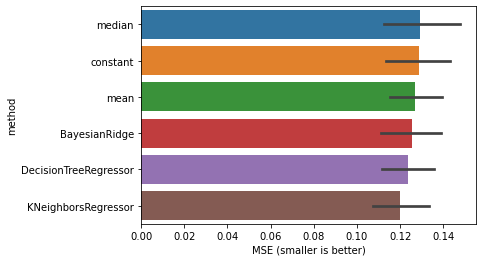The plot above presents the result of the 5-fold cross-validation reshuffled and repeated 5 times for each estimator. Note that by considering the above cross-validation we are assuming that samples should be more similar to others belonging to the same class. All methods show a somewhat similar MSE ($0.12 \lessapprox \overline{MSE} \lessapprox 0.13$). However, we actually have the complete dataset with no missing data :) So we can benchmark the different imputation methods above by computing the MSE or RMSE against the complete dataset for each imputation method. In : ### Complete data complete_gex=pd.read_csv(data_dir+'gene_expression_complete.tsv', sep="\t", index_col=0) complete_gex=complete_gex.loc[:,~complete_gex.columns.isin(['ensembl','gene_description'])] complete_gex.columns=metadata.reset_index().set_index('samples').loc[ [x for x in complete_gex.columns.values if x in metadata['samples'].values],'group_sample'] complete_gex=complete_gex.loc[gex_filtered.index, gex_filtered.columns] # standardize complete dataset complete_gex_std=pd.DataFrame(sklearn.preprocessing.StandardScaler().fit_transform(complete_gex.T), index=complete_gex.columns, columns=complete_gex.index).T # dictionary with all imputed datasets df_dict={ 'constant_0':imputed_GEX_0, 'row_mean':imputed_GEX_rowMEAN, 'row_median':imputed_GEX_rowMEDIAN, 'row_min':imputed_GEX_rowMIN, 'group_mean':imputed_GEX_groupMEAN, 'group_median':imputed_GEX_groupMEDIAN, 'group_min':imputed_GEX_groupMIN, 'group_min95':imputed_GEX_groupMIN95, 'knn':imputed_GEX_KNN, 'knn8':imputed_GEX_KNN8, 'bayesian_ridge':imputed_GEX_bayesianr, 'decision_trees':imputed_GEX_trees } imputation_mse=pd.DataFrame() for imp_method in df_dict.keys(): if(imp_method in ['knn', 'knn8','bayesian_ridge', 'decision_trees']): complete_cases=complete_gex_std #use standardized data for KNN, BayesianRidge or DecisionTrees else: complete_cases=complete_gex mse=((df_dict[imp_method] - complete_cases)**2).mean().mean() rmse=np.sqrt(mse) imputation_mse[imp_method]=pd.Series([mse,rmse],index=['MSE','RMSE']) imputation_mse=imputation_mse.T.reset_index().rename(columns={'index':'imputation_method'}).sort_values('MSE',ascending=False) imputation_mse['strategy']=['multivariate' if x in ['bayesian_ridge','decision_trees'] else 'univariate' for x in imputation_mse.imputation_method] fig,ax = plt.subplots(figsize=(10,6), ncols=2, sharey=True) ax=ax.flatten() sb.barplot( data=imputation_mse, x='MSE', y='imputation_method', hue='strategy', dodge=False, ax=ax ); ax.set(xscale='log'); sb.barplot( data=imputation_mse, x='RMSE', y='imputation_method', hue='strategy', dodge=False, ax=ax ); ax.set(xscale='log', ylabel=''); fig.suptitle('Imputation error (sorted, lower is better)'); fig.tight_layout()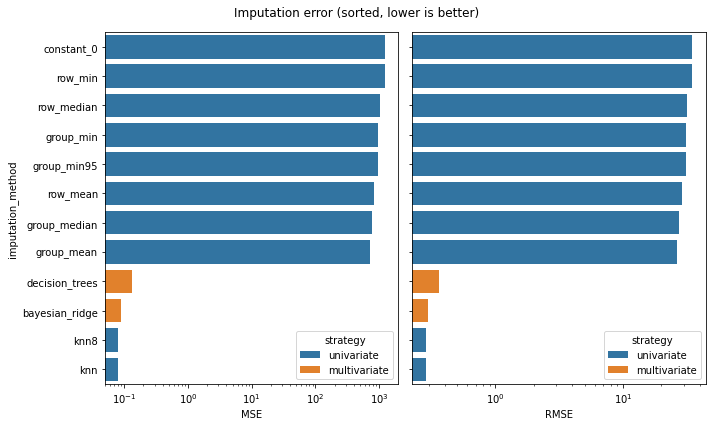It is interesting to see that in here, just like in the cross-validated approach above, the KNN, Bayesian Ridge and Decision Trees imputers show the lowest error, but now substantially lower than any other methods. The magnitude difference in MSE in here against the cross-validated set above is probably due to our using the full (complete) dataset in here instead of a cross-validated one. Group mean / median imputers show a slightly lower error than row-wise imputation methods, though imputation by row_means is still favored against minimum imputation by group. For this particular case, one can also highlight that multivariate imputation does not present an advantage over univariate methods given that KNN, Bayesian Ridge and Decision Trees all display similar error. While the above working example highlights KNN as a the best imputation method, which has also been observed by others, the choice of best imputation method should be examined for each case independently. Note that many other imputation methods can be used. An analysis of 10 different data imputation methods in a microarray cancer dataset shows similar NRMSE irrespective of the method that is applied. However, this may depend on different scenarios of missigness, and from dataset to dataset. For instance, analysis of 5 different machine learning datasets points to KNN as the imputation method that shows the lowest overall NRMSE. An analysis of imputation methods in proteomics indicates that no rull-of-thumb can be derived in general, but that the best imputation method should be identified for each dataset. You can read more about imputation in this introduction, here, and here. For instance, a comparison between KNN, SVD and row mean as imputation method was performed here. A practical and more comprehensive guide about data imputation in R can be found here. ## anomaly detection¶ When cleaning our data we often need to identify observations that do not conform to the behavior of the data. Due to sampling errors or technical biases in our experiment, some observations may behave substantially differently than the rest of our data. Note that identifying outliers is different than novelty detection that aims to identify whether a new observation behaves similarly to our data, and as such can use a trained model to test whether a given observation is considered as novel. In this section we focus on outlier identification at the sample level, and leave feature selection for another part of our course. In identifying outliers, we usually consider unsupervised approaches and that there are substantially more observations that behave "normally" than "abnormally". We usually approach the outlier detection problem by building a profile of what "normally"-behaving dataset looks like (e.g. gaussian distribution), and then applying a statistical test to measure how "far" (likelihood) an observation is from the given observation. We need to define how many features we will use to identify them (univariate vs multivariate), and whether we can assume a data distribution (parametric vs non-parametric). Here we will look at: Note that any other approaches can be used for outlier detection - for instance, you can have a look at Random Cut Forest, the algorithm employed by Amazon. In this section we will only employ outlier detection at the sample level, but one could possible use these approaches together with dimensionality reduction to reduce the number of features in our dataset. In : # Imputed data without standardization for later use imputed_KNN_raw=pd.DataFrame( KNNImputer(n_neighbors=2).fit_transform(gex_filtered.T), index=gex_filtered.columns, columns=gex_filtered.index).T # Imputation by KNN imputed_GEX_KNN_metadata=imputed_GEX_KNN.copy().T.merge(metadata.copy(), left_index=True, right_index=True) ## data with added sample annotations ## collecting the results of the different outlier detection methods all_outliers=pd.DataFrame(columns=['outlier','method'])  ### Descriptive statistics and preliminary visual inspection¶ In case we had univariate or bivariate data, we could inspect our dataset with simple scatterplots to give us a sense to possible outliers. We will do so by examining two random genes, DHODH and RIDA. The scatterplot (left) shows that while most samples are clustering together, some display very high DHODH / RIDA expression (left plot), and the Z-score distributions of these samples could point towards potential outliers such as those samples showing Z > 2 (i.e. >2$\sigma$, containing$\approx$95.4% of the population), thus away from the population mean (elipses, center plot). However, if we look at sample classes (right plot), we notice that most of the samples showing Z > 2 belong to the control group, so it is probably not a good idea to drop these samples. If we considered the traditional threshold of$3 \sigma$, we still observe that most of the samples identified as outliers belong to the control group. Note however that this approach assumes that all samples are derived from the same population, and that data is normally distributed. We can already see that this is not the case, as samples seem to be clustering by control vs disease group1/group2. In : fig,ax=plt.subplots(figsize=(16,8), ncols=2, sharey=True) ax=ax.flatten() #z-scores sb.scatterplot( data=pd.DataFrame( sp.stats.zscore(imputed_GEX_KNN_metadata[['DHODH','RIDA']].values), index=imputed_GEX_KNN_metadata.index, columns=['DHODH','RIDA'] ).join(imputed_GEX_KNN_metadata['group']), x='DHODH', y='RIDA', ax=ax); #z-scores w/groups sb.scatterplot( data=pd.DataFrame( sp.stats.zscore(imputed_GEX_KNN_metadata[['DHODH','RIDA']].values), index=imputed_GEX_KNN_metadata.index, columns=['DHODH','RIDA'] ).join(imputed_GEX_KNN_metadata['group']), x='DHODH', y='RIDA', hue='group', ax=ax); #text labels for diam in [2,4,6]: for axi in [0,1]: ax[axi].add_artist(matplotlib.patches.Ellipse((0, 0), diam, diam,edgecolor='gray',facecolor='none')) ax[axi].text((diam/2)+0.05, 0, "Z = "+str(int(diam/2)), ha="left", va="top") # ax.title.set_text('Gene expression') ax.title.set_text('Z-scores') ax.title.set_text('Z-scores w/groups')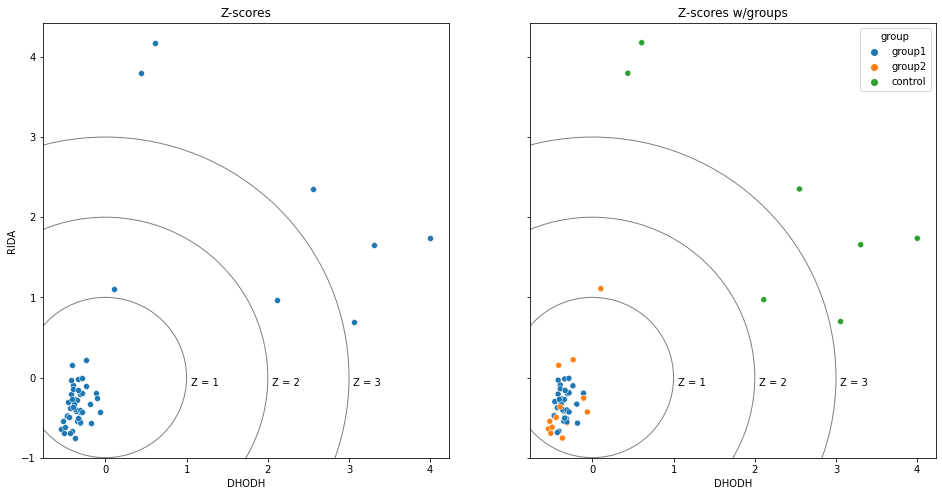Often we also see outlier identification with respect to interquartile ranges (IQR). These are traditionally defined as $$x \notin [\text{Q1} - 1.5 \times \text{IQR}, \text{Q3} + 1.5 \times\text{IQR}]$$ This range is slightly below$\pm 3 \sigma$that contains the top/bottom 0.15% of the observations). Below we define the regions that would find the inliers (i.e. those within the range above) for the full dataset (black box), as well for each group. Again we see that all control points would be identified as outliers if we considered the entire dataset (black box). Note however the large region defined by the control group (green box). In : quantile_df=pd.DataFrame(index=['control','group1','group2','full']) for gene in ['DHODH','RIDA']: for quant in [0.25,0.75]: quantile_df=quantile_df.join( imputed_GEX_KNN_metadata[[gene,'group']].groupby('group')[gene].agg(lambda x: np.quantile(x, quant)).rename(gene+'_'+str(quant))) quantile_df.loc['full',gene+'_'+str(quant)]=imputed_GEX_KNN_metadata[[gene]].quantile(quant).values quantile_df['DHODH_IQR']=quantile_df['DHODH_0.75']-quantile_df['DHODH_0.25'] quantile_df['RIDA_IQR']=quantile_df['RIDA_0.75']-quantile_df['RIDA_0.25'] quantile_df['DHODH_lower_lim']=quantile_df['DHODH_0.25']-1.5*quantile_df['DHODH_IQR'] quantile_df['RIDA_lower_lim']=quantile_df['RIDA_0.25']-1.5*quantile_df['RIDA_IQR'] quantile_df['DHODH_upper_lim']=quantile_df['DHODH_0.75']+1.5*quantile_df['DHODH_IQR'] quantile_df['RIDA_upper_lim']=quantile_df['RIDA_0.75']+1.5*quantile_df['RIDA_IQR'] fig,ax=plt.subplots(figsize=(16,5)) ### IQR of the full dataset for i in range(4): groupi=['full','group1','group2','control'][i] ax.add_patch(matplotlib.patches.Rectangle( (quantile_df.loc[groupi,'DHODH_lower_lim'], quantile_df.loc[groupi,'RIDA_lower_lim']), quantile_df.loc[groupi,'DHODH_upper_lim']-quantile_df.loc[groupi,'DHODH_lower_lim'], quantile_df.loc[groupi,'RIDA_upper_lim']-quantile_df.loc[groupi,'RIDA_lower_lim'], fill=False, color=['k','blue','orange','green'][i], linewidth=2.5, alpha=0.6 )) #full data sb.scatterplot( data=imputed_GEX_KNN_metadata, x='DHODH', y='RIDA', hue='group', ax=ax); ax.title.set_text('Gene expression (boxes give inlier regions)')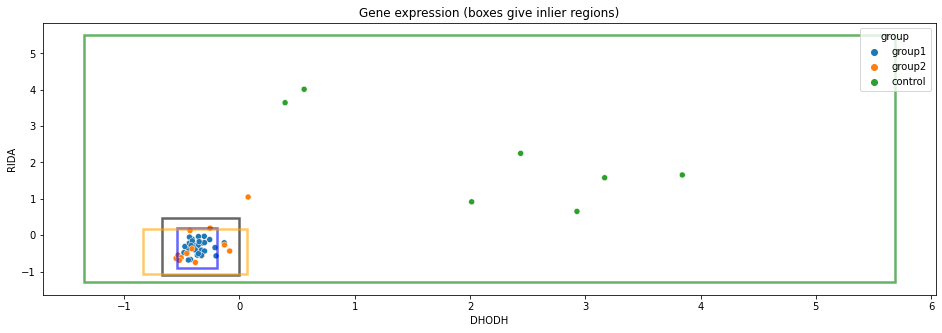The above definition of outliers is actually used for identification of outliers when drawing boxplots. In the next figure, we plot the sample values for the two genes. Notice the dots, identified as outliers in each distribution, and the much lower IQR for the full set (gray) than for the control group (green). The outliers identified for the full dataset correspond to those in the control group as we have seen above. In : ### We create one dummy df so that we can see the distribution of the samples based on the full dataset full_ds=imputed_GEX_KNN_metadata[['DHODH','RIDA']].unstack().reset_index().rename(columns={'level_0':'gene','level_1':'samples',0:'expression'}).merge(metadata['group'], left_on='samples', right_index=True) dict_colors={'group1':'blue','group2':'darkorange','control':'green','full':'gray'} dummy_ds=full_ds.copy() dummy_ds.loc[:,'group']='full' full_ds=pd.concat([full_ds, dummy_ds]) fig,ax=plt.subplots(figsize=(16,5)) g=sb.boxplot(data=full_ds,x='expression', y='gene', hue='group', palette=dict_colors) g.legend(loc='center right', bbox_to_anchor=(1, 0.87), ncol=1); #change legend location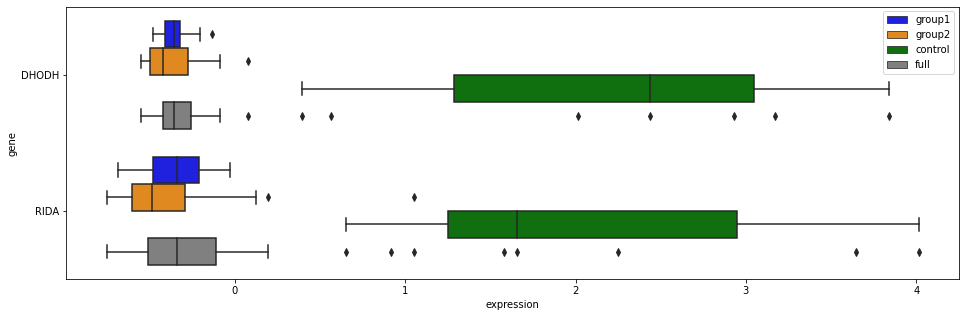However, since we often need to deal with multivariate data, we can have an initial idea of how similar the samples are using pairwise sample correlations based on the entire feature set. In : row_order=imputed_GEX_KNN_metadata.sort_values('group').index.values col_colors=[dict_colors[x] for x in imputed_GEX_KNN_metadata.loc[imputed_GEX_KNN_metadata.sort_values('group').index.values, 'group']] g=sb.clustermap( imputed_GEX_KNN.corr(method='spearman').loc[row_order, row_order], row_cluster=True, col_cluster=True, metric='euclidean', method='centroid', row_colors=col_colors, col_colors=col_colors, linewidths=0, xticklabels=False, yticklabels=False, center=0, cmap='seismic' ) #legend for label in imputed_GEX_KNN_metadata['group'].unique(): g.ax_col_dendrogram.bar(0, 0, color=dict_colors[label], label=label, linewidth=0) g.ax_col_dendrogram.legend(loc="upper right", ncol=6);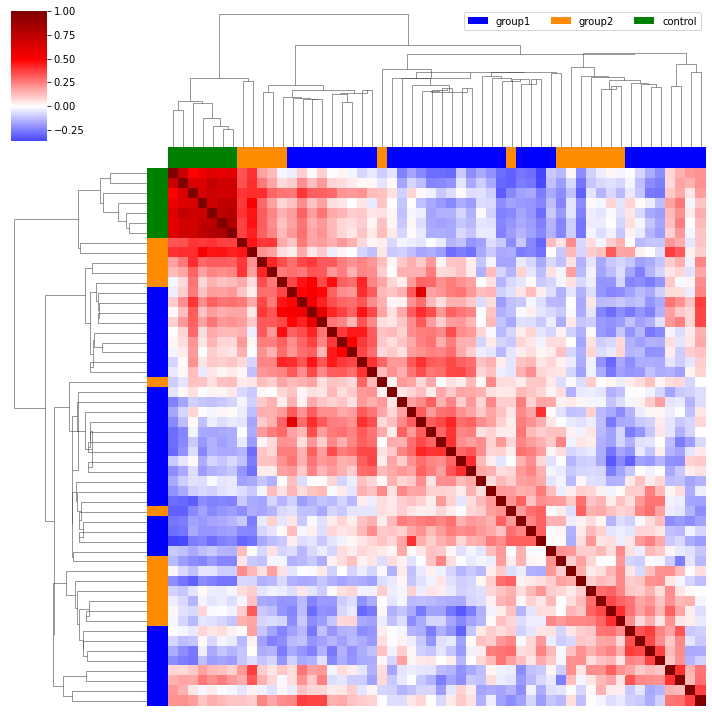In the heatmap above we used Euclidean distance and centroid as linkage method, but what would happen if we used other metrics or linkage methods? You can read more about different distance and linkage metrics here. Note how the hiearchical clustering shows that control samples are very close to each other, but that a group of samples belonging to group 2 are clustering more closely with the controls than with their respective groups. Can we consider them as outliers? What if looked at sample relationships in 2 dimensions, similarly to how we used 2 random genes above, but instead finding a representation that would explain as much variability in our data as possible as possible? This is what we will do through principal component analysis. Outliers increase classic measures of variance of a dataset, and given that principal components follow the directions of maximum variance, a PCA is sensitive to outliers. We will further use the first 2 PCs to identify observation distance to model in X-space, or distance to the PCs. This metric briefly takes into consideration each observation's residuals against the model's residuals to compute the amount of variation that is unexplained by the model plane (see this for more information). Observations exceeding the critical distance in DModX (typically 0.05) do not fit the model well and are considered outliers. Other metrics include Hotelling's$T^2\$ method, not examined here.

We will now plot the dataset above based on the first 2 PCs. Note that we have previously standardized the data, and data scaling is crucial for PCA. Scikitlearn has all the necessary tools to perform PCA (example), but to facilitate plotting of different metrics, we use the pca package (which uses scikitlearn on the backend).

In :
## setting group as row labels for plotting

model=pca(
n_components=0.95,
alpha=0.05, # alpha for detecting outliers
normalize=False #data had been standardized before
)
results=model.fit_transform(group_labeled_rows, verbose=0)

model.scatter();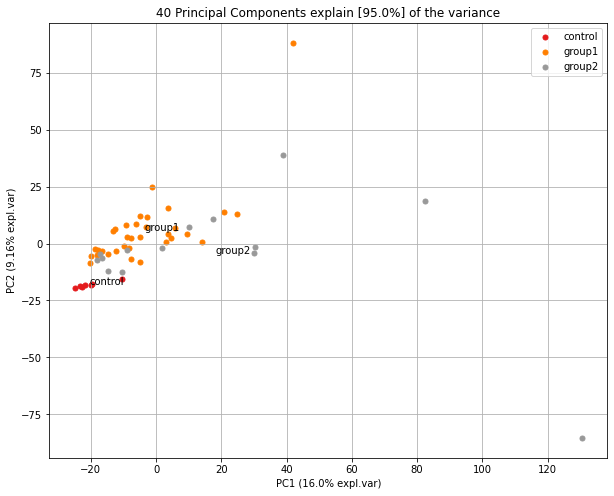Note in the plot above how control samples are clustering very closely, just like most of those in groups 1 and 2. However, a few samples lie far away from most of the population, and they would be identified as outliers if we were to consider the distance to the PCA plane. The following figure highlights the elipse defined by DModX, with outliers now marked as diamonds.

In :
model.scatter(SPE=True);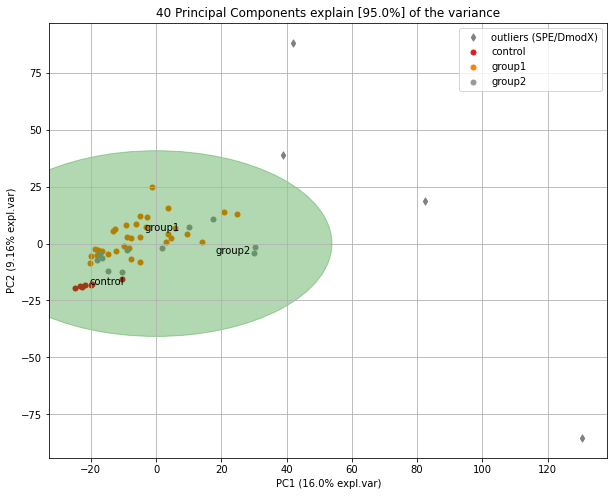We will re-run the code after dropping the samples identified as outliers.

In :
model=pca(n_components=0.95, alpha=0.05, normalize=False);
results=model.fit_transform(imputed_GEX_KNN.T, verbose=0);

### Outliers with sample labels
outlier_list=results['outliers']
outlier_list=outlier_list.loc[outlier_list.y_bool_spe==True].index.values
print(f'Outlier samples removed:\n\t{outlier_list}')

## Retrieving sample names as outliers
## and adding them to the all_outliers dataframe for later inspection
outliers_ellipse=pd.DataFrame(
[
model.results['outliers'].loc[model.results['outliers']['y_bool_spe']==True,].index,
np.repeat(['PCA_ellipse'], len(model.results['outliers'].loc[model.results['outliers']['y_bool_spe']==True,].index))
], index=['outlier','method']).T

all_outliers=pd.concat([all_outliers, outliers_ellipse])

### only inliers
# setting group as row labels for plotting
inlier_list=imputed_GEX_KNN.copy().columns.values[~imputed_GEX_KNN.columns.isin(outlier_list)]

# modeling only on inliers
model=pca( n_components=0.95, alpha=0.05, normalize=False)
results=model.fit_transform(group_labeled_rows_inliers, verbose=0)

model.scatter();

Outlier samples removed:
['sample_10.g1' 'sample_36.g2' 'sample_42.g2' 'sample_43.g2']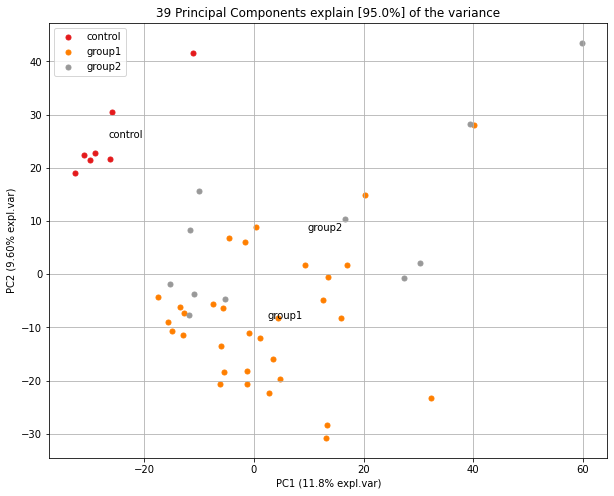We see above that the top 2 PCs show a much more homogeneous sample distribution after removing the samples identified as outliers. The approaches above assume normally-distributed data, which is not always the case.

### HDBSCAN¶

The Density-Based Clustering Based on Hierarchical Density Estimates (HDBSCAN) is an extension to DBSCAN (demo). Briefly, this clustering method identifies clusters based on areas with high density of observations, sepparated by areas with low density, and extracts clusters based on their stability. To do so, this algorithm uses the minimum sample and minimum cluster size hyperparameters, together with sample distance metrics, to identify core points (those at the center of a cluster), border points (those near the border of a cluster), and noise points (those not belonging to a cluster, and considered as anomalies). More information and demonstration of how HDBSCAN works can be found in here. There is some hyperparameter tuning to be done that we will not cover here.

Let's examine how the outlier scores when setting the minimum size of a cluster to 2 or 4.

In :
for min_clust in [2,4]:
clusterer = hdbscan.HDBSCAN(min_cluster_size=min_clust).fit(imputed_GEX_KNN.T)
sb.displot(clusterer.outlier_scores_[np.isfinite(clusterer.outlier_scores_)], rug=True, kde=True, bins=15)
plt.title('HDBSCAN outlier score (min_cluster_size = '+str(min_clust)+')');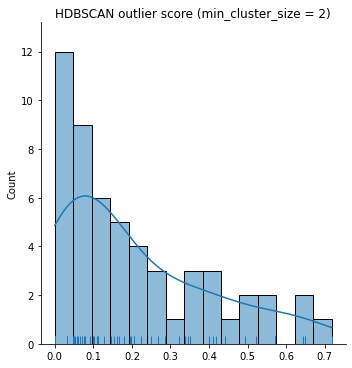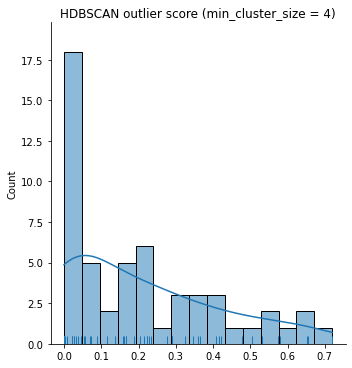Note how increasing min_cluster_size leads to a higher number of points with lower outlier scores. We will consider that min_cluster_size = 4 based on the sample similarity that we computed above (through KNN in the Imputation section). In the next cell we explore different quantile thresholds for identifying outliers.

In :
for quantile_val in [0.7, 0.95]:
clusterer = hdbscan.HDBSCAN(min_cluster_size=4).fit(imputed_GEX_KNN.T)
threshold = pd.Series(clusterer.outlier_scores_).quantile(quantile_val)
outliers = np.where(clusterer.outlier_scores_ > threshold)
print(f'Quantile: {quantile_val}   | Number of outliers: {len(outliers)}')

## setting group as row labels for plotting

# adding outliers to summary table
outlier_list=[outlier_labeled_rows.index[x] for x in range(outlier_labeled_rows.shape) if (x in outliers)]
all_outliers=pd.concat([all_outliers,
pd.DataFrame([outlier_list, np.repeat('Quantile_'+str(quantile_val),len(outlier_list))], index=['outlier','method']).T])

# label outliers
outlier_labeled_rows.set_index('group', inplace=True)
outlier_labeled_rows.index=[outlier_labeled_rows.index[x] if (x not in outliers) else 'outlier' for x in range(outlier_labeled_rows.shape)]

model=pca(n_components=0.95, normalize=False)
results=model.fit_transform(outlier_labeled_rows, verbose=0)
model.scatter(cmap='tab20');

Quantile: 0.7   | Number of outliers: 16
Quantile: 0.95   | Number of outliers: 3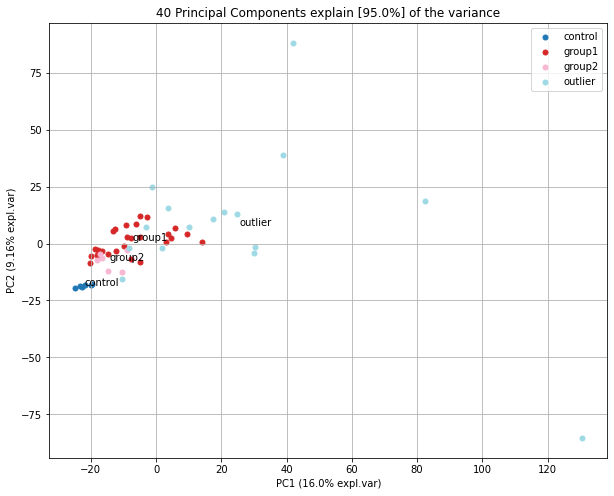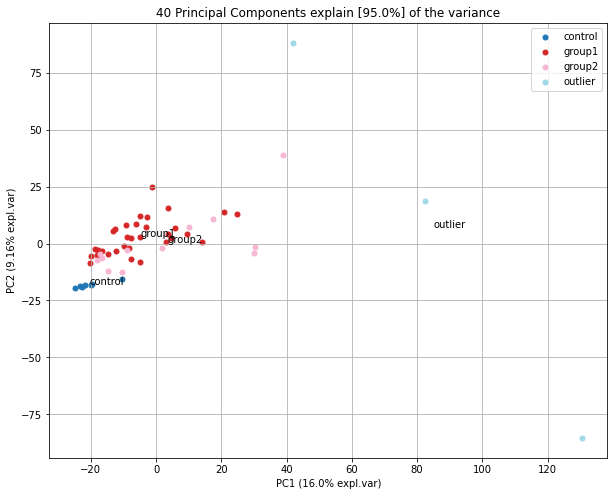Note in the 1st PCA above (quantile 0.7) that sparse samples tend to be identified as outliers, but those similar in the 2 PC-space are not labeled as outliers. When considering as outliers those points above the 95% quantile, we still see that points identified as outliers are those 3 group1/group2 samples that show the highest sparsity.

We have not explored other hyperparameters of HDBSCAN such as min_samples (the number of samples in a neighborhood, controls how tolerant the algorithm is to noise), metric (the distance metric), or cluster_selection_epsilon (the distance threshold for merging clusters). You can explore how these parameters influence clustering in here.

### Local Outlier Factor¶

The Local Outlier Factor (LOF) is a method with some commonalities to DBSCAN. LOF also seeks to compare density with respect to a given sample and compare it with the densities of samples in its neighbours, to determine which samples have a lower density compared to their neighbors, and as such should be considered as outlier. In this method, the "local density" is estimated based on the number of samples in a given neighborhood, and depends on the hyperparameter n_neighbors.

Below, we also consider euclidean distance as metric, and set outliers to represent 5% of the samples.

In :
def LOF_PCA(n_neighbor, contamination, pc1=0, pc2=1):
"""
A function to perform LOF and represent the resulting outliers in PCA space (PC1 vs PC2)
"""
LOF=sklearn.neighbors.LocalOutlierFactor(
n_neighbors=n_neighbor,
metric='euclidean',
n_jobs=-1,
contamination=contamination #fraction of samples to consider as outliers
)
results=LOF.fit(imputed_GEX_KNN.T)
outliers=np.where(results.fit_predict(imputed_GEX_KNN.T) == -1)

print(f'n_neighbors: {n_neighbor} | outlier fraction: {contamination} | Number of outliers: {len(outliers)}')

## setting group as row labels for plotting

# adding outliers to summary table
outlier_list=[outlier_labeled_rows.index[x] for x in range(outlier_labeled_rows.shape) if (x in outliers)]
outlier_df=pd.DataFrame([outlier_list, np.repeat('LOF_contamination'+str(contamination)+'_k'+str(n_neighbor),len(outlier_list))], index=['outlier','method']).T

# label outliers
outlier_labeled_rows.set_index('group', inplace=True)
outlier_labeled_rows.index=[outlier_labeled_rows.index[x] if (x not in outliers) else 'outlier' for x in range(outlier_labeled_rows.shape)]

model=pca(n_components=0.95, normalize=False)
results=model.fit_transform(outlier_labeled_rows, verbose=0)
model.scatter(cmap='tab20', PC=[pc1,pc2])

return(outlier_df)

# we plot and add the list of outliers to the summary DF
all_outliers=pd.concat([all_outliers, LOF_PCA(n_neighbor=4, contamination=0.05)])

n_neighbors: 4 | outlier fraction: 0.05 | Number of outliers: 3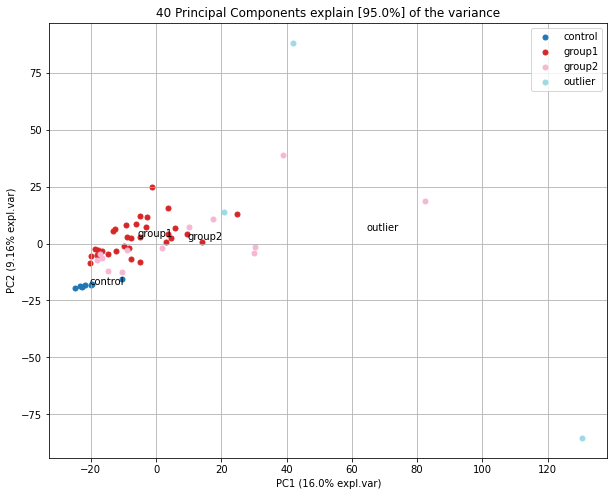Curiously, the first two plots further define a point in the center as outlier. Can you hypothesize why? (hint: pc3 and pc4)

If we increased the outlier ratio to 30%, we would see that the most sparse points at the top 2 PCs are identified as outliers.

In :
all_outliers=pd.concat([all_outliers, LOF_PCA(n_neighbor=4, contamination=0.3)])

n_neighbors: 4 | outlier fraction: 0.3 | Number of outliers: 16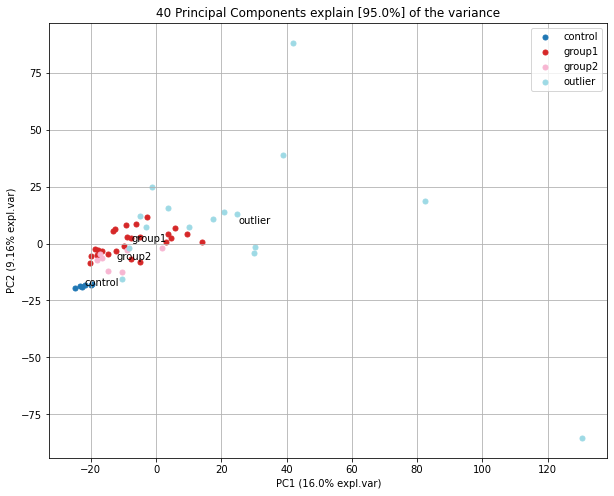This is even clearer if we look at PCs 4 and 5 where samples away from control and groups 1 and 2 are flagged as outliers.

In :
LOF_PCA(n_neighbor=4, contamination=0.3, pc1=4, pc2=3);

n_neighbors: 4 | outlier fraction: 0.3 | Number of outliers: 16